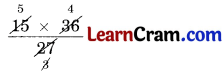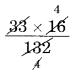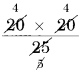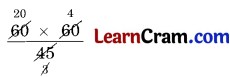# DAV Class 6 Maths Chapter 4 Worksheet 2 Solutions

The DAV Class 6 Maths Book Solutions Pdf and DAV Class 6 Maths Chapter 4 Worksheet 2 Solutions of Ratio, Proportion and Unitary Method offer comprehensive answers to textbook questions.

## DAV Class 6 Maths Ch 4 WS 2 Solutions

Question 1.
Are the following numbers in proportion?

(a) 9, 12, 18, 24
Solution:
9, 12, 18, 24
Product of extremes = 9 × 24 = 216
Product of means = 12 × 18 = 216
Hence, 9 : 12 : : 18 : 24.

(b) 3, 4, 8, 16
Solution:
3, 4, 8, 16
Product of extremes = 3 × 16 = 48
Product of means = 4 × 8 = 32
Product of extremes is not equal to the product of means
∴ 3, 4, 8, 16 are not in proportion.(c) 22, 33, 16, 24
Solution:
22, 33, 16, 24
Product of extremes = 22 × 24 = 528
Product of means = 33 × 16 = 528
Hence, 22 : 33 : : 16 : 24.

(d) 63, 55, 32, 72
Solution:
63, 55, 32, 72
Product of extremes = 63 × 72 = 4536
Product of means = 55 × 32 = 1760
Product of extremes is not equal to product of means
∴ 63, 55, 32, 72 are not in proportion.

(e) 4, 9, 32, 72
Solution:
4, 9, 32, 72
Product of extremes = 4 × 72 = 288
Product of means = 9 × 32 = 288
Hence, 4 : 9 : : 32 : 72.

(f) 15, 75, 45, 120
Solution:
15, 75, 45, 120
Product of extremes = 15 × 120 = 1800
Product of means = 75 × 45 = 3375
Product of extremes is not equal to product of means
∴ 15, 25, 45, 120 are not in proportion.Question 2.
Fill in the following boxes so that the four numbers are in proportion.

(a) 32, ___, 6, 12
Solution:
32, ___, 6, 12
If the given numbers are in proportion
∴ 32 : ___ : : 6 : 12
___ × 6 = 32 × 12
___ = $$\frac{32 \times 12}{6}$$
___ = 64
Hence, 64 should be filled in the blank box.

(b) 21, 27, 14, ___
Solution:
21, 27, 14, ___
If the given numbers are in proportion
∴ 21 : 27 : : 14 : ___
___ × 21 = 27 × 14
___ =Hence, the required number = 18.

(c) 15, ___, 27, 36
Solution:
15, ___, 27, 36
If the give numbers are in proportion
∴ 15 : ___ : : 27 : 36
___ × 27 = 15 × 36
___ =Hence, the required number = 20.

(d) 33, 132, ___, 16
Solution:
33, 132, ___, 16
If the given numbers are in proportion
∴ 33 : 132 : : ___ : 16
___ × 132 = 33 × 16
___ == 4
Hence, the required number = 4.Question 3.
Are the following in continued proportion?

(a) 27, 36, 42
Solution:
27, 36, 42
If they are in continued proportion
∴ 27 : 36 : : 36 : 24
Product of extremes = 27 × 24 = 648
Product of means = 36 × 36 = 1296
∴ 648 ≠ 1296
Hence, they are not in continued proportion.

(b) 3, 9, 27
Solution:
3, 9, 27
If they are in continued proportion
∴ 3 : 9 : : 9 : 27
Product of extremes = 3 × 27 = 81
Product of means = 9 × 9 = 81
Hence, they are in continued proportion.

(c) 48, 36, 27
Solution:
48, 36, 27
If they are in continued proportion
∴ 48 : 36 : : 36 : 27
Product of extremes = 48 × 27 = 1296
Product of means = 36 × 36 = 1296
Hence, they are in continued proportion.

(d) 36, 90, 75
Solution:
36, 90, 75
They are in continued proportion
If 36 : 90 : : 90 : 75
Product of extremes = 36 × 75 = 2700
Product of means = 90 × 90 = 8100
∴ 2700 ≠ 8100
Hence, they are not in continued proportion.Question 4.
Fill in the boxes so that the three numbers are in continued proportion:

(a) 6, 18, ___
Solution:
6, 18, ___
As they are in continued proportion
∴ 6 : 18 : : 18 : ___
6 × ___ = 18 × 18
___ == 54
Hence, the required number = 54.

(b) 25, 20, ___
Solution:
25, 20, ___
As they are in continued proportion
∴ 25 : 20 : : 20 : ___
25 × ___ = 20 × 20
___ == 16
Hence, the required number = 16.

(c) ___, 32, 64
Solution:
___, 32, 64
As they are in continued proportion
∴ ___ : 32 : : 32 : 64
___ × 64 = 32 × 32
___ == 16
Hence, the required number = 16.

(d) ___, 60, 45
Solution:
___, 60, 45
As they are in continued proportion
∴ ___ : 60 : : 60 : 45
___ × 45 = 60 × 60
___ == 80
Hence, the required number = 80.Question 5.
Determine if the following ratios are in proportion:

(a) 48 kg : 6 kg and 25 g : 200 g
Solution:
48 kg : 6 kg and 25 g : 200 g
They are in proportion if 48 : 6 : : 25 : 200
Product of extremes = 48 × 200 = 9600
Product of means = 6 × 25 = 150
9600 ≠ 150
Hence, they are not in proportion.

(b) 8 m : 21 m and ₹ 24 : ₹ 63
Solution:
8 m : 21 m and ₹ 24 : ₹ 63
They are in proportion if 8 : 21 : : 24 : 63
Product of extremes = 8 × 63 = 504
Product of means = 21 × 24 = 504
Hence, they are in proportion.

(c) 45 girls : 60 girls and 48 boys : 64 boys
Solution:
45 girls : 60 girls and 48 boys : 64 boys
They are in proportion
if 45 : 60 : : 48 : 64
Product of extremes = 45 × 64 = 2880
Product of means = 60 × 48 = 2880
Hence, they are in proportion.

(d) 5.2 l : 3.9 l = 3 ml : 4 ml
Solution:
They are in proportion
if 5.2 : 3.9 : : 3 : 4
Product of extremes = 5.2 × 4 = 20.8
Product of means = 3.9 × 3 = 11.7
20.8 ≠ 11.7
Hence, they are not in proportion.Question 6.
Fill in the following blanks:

(a) Equality of two ratios is called _________.
Solution:
Proportion

(b) A proportion has _________ terms.
Solution:
4

(c) The first and fourth terms of a proportion are called _________.
Solution:
extremes

(d) The _________ and _________ terms of a proportion are called means.
Solution:
second, third

(e) For 4 numbers to be in proportion, the product of _________ should be equal to the product of _________.
Solution:
extremes, means